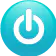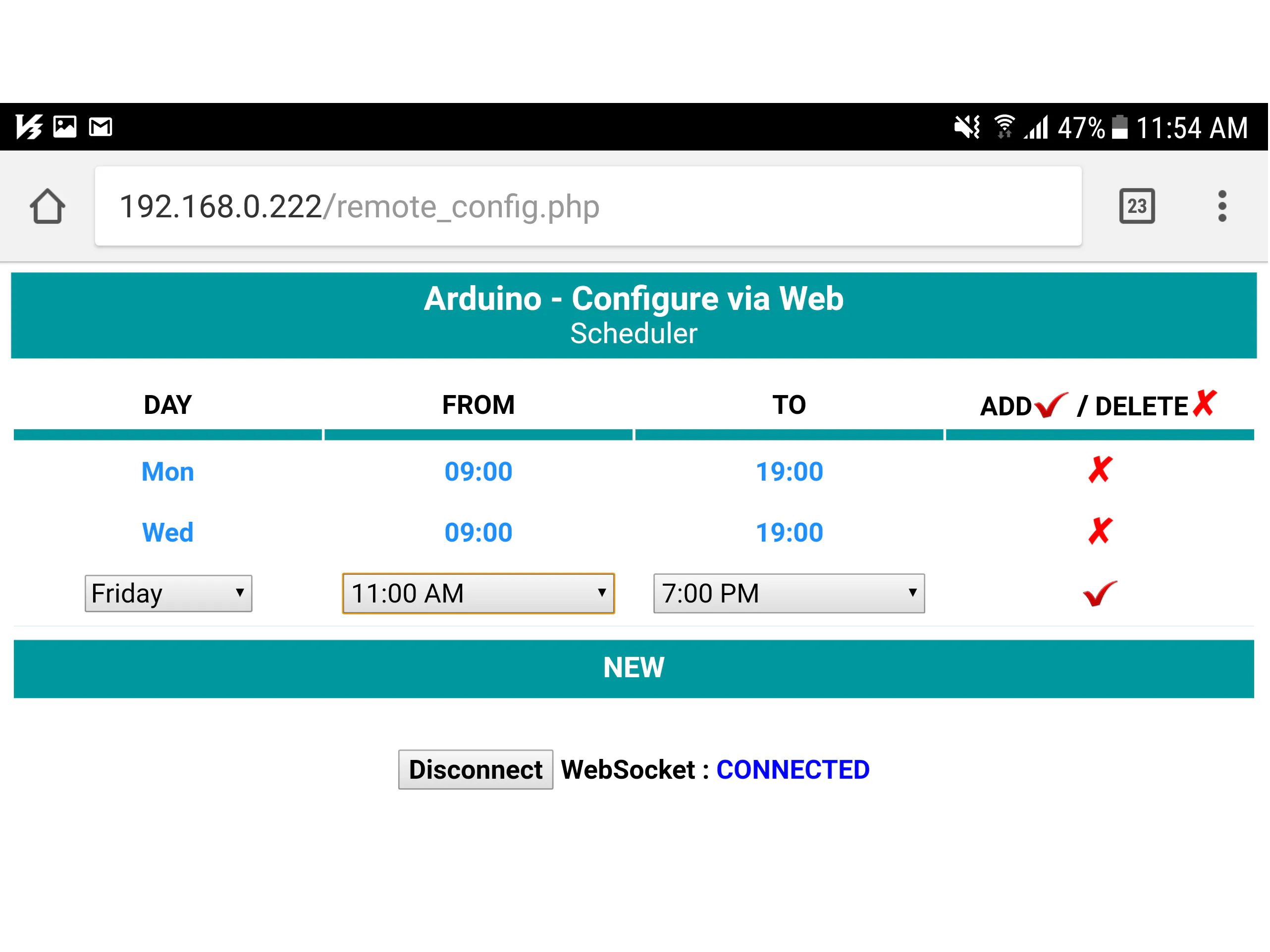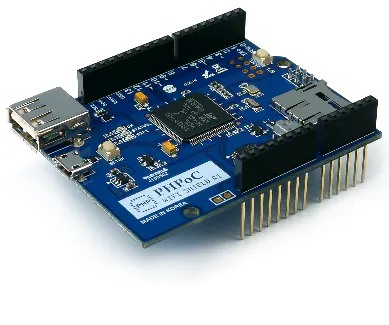# Arduino - Set Schedule via Web

The project shows you how to configure flexibly user variables via Web without hard-coding. Setting Schedule via web is an example.

IntermediateFull instructions provided2,941## Things used in this project

### Hardware componentsArduino UNO & Genuino UNO
×1PHPoC WiFi Shield for Arduino
×1
 LED
×1

## Code

### WebConfigSchedule.ino

Arduino
This is Arduino code.
```#include "SPI.h"
#include "Phpoc.h"
#include <EEPROM.h>

#ifndef EOF
#define EOF (-1)
#endif

#define CMD_ARDUINO_CLR 0
#define CMD_ARDUINO_GET 1
#define CMD_WEB_CLR		5
#define CMD_WEB_UPD		6

#define MAX_SCHEDULE	10

#define	DAY				0
#define START_HOUR		1
#define START_MINUTE	2
#define END_HOUR		3
#define END_MINUTE		4

class EepromCSV
{
public:
void clear(void);
int line(void);
void writeLine(String lineStr);
};

EepromCSV EepromCSV;
PhpocServer configServer(80);
PhpocDateTime datetime;

byte scheduleArray[MAX_SCHEDULE];
int numSchedule;

/* Varalble to blink a LED*/
const int ledPin = 7;
int ledState = LOW;
unsigned long previousMillis = 0;
const long interval = 500;

void setup() {
Serial.begin(9600);
while(!Serial)
;
Phpoc.begin(PF_LOG_SPI | PF_LOG_NET);
configServer.beginWebSocket("arduino_config");

Serial.println(Phpoc.localIP());

eepromToSchedule(); // load schedule from EEPROM

pinMode(ledPin, OUTPUT);
}

void loop() {
scheduleLoop(); // check whether there is any config command from Web or not.

if(isOnSchedule()) { // check whether current time is on schedule or not
/* TO DO */
unsigned long currentMillis = millis();

if(currentMillis - previousMillis >= interval) {
previousMillis = currentMillis;

if (ledState == LOW)
ledState = HIGH;
else
ledState = LOW;

digitalWrite(ledPin, ledState);
}
}
else {
if (ledState == HIGH) {
ledState = LOW;
digitalWrite(ledPin, ledState);
}
}
}

void eepromToSchedule(void) {
String schedule;
int lineCount = EepromCSV.line();

if(lineCount > MAX_SCHEDULE)
lineCount = MAX_SCHEDULE;

for(int i = 0; i < lineCount; i++) {

scheduleArray[i][DAY]			= schedule.substring(0, 1).toInt();
scheduleArray[i][START_HOUR]	= schedule.substring(2, 4).toInt();
scheduleArray[i][START_MINUTE]	= schedule.substring(5, 7).toInt();
scheduleArray[i][END_HOUR]		= schedule.substring(8, 10).toInt();
scheduleArray[i][END_MINUTE]	= schedule.substring(11, 13).toInt();
}

numSchedule = lineCount;
}
void sendToWeb(String data) {
char wbuf;

data.toCharArray(wbuf, data.length() + 1);
configServer.write(wbuf, data.length());
}
void scheduleLoop(void) {
PhpocClient client = configServer.available();

if(client) {
if(client.available() >= 17) {
char rbuf;
String data, schedule;
int cmd;

data = String(rbuf);
cmd = data.substring(0, 1).toInt();

if(cmd == CMD_ARDUINO_GET) {
int lineCount = EepromCSV.line();

sendToWeb(String(CMD_WEB_CLR) + ",0,00:00,00:00\r\n"); /* tell web clear to load new schedule */

for(int i = 0; i < lineCount; i++) {
schedule = EepromCSV.readLine(i); /* read schedule from EEPROM one by one */
sendToWeb(String(CMD_WEB_UPD) + "," + schedule + "\r\n"); /* send schedule to Web */
}
}
else if(cmd == CMD_ARDUINO_CLR) {
EepromCSV.clear(); /* clear to update new schedule */
eepromToSchedule(); /* reload schedule from EEPROM */
}
schedule = data.substring(2, 15);
EepromCSV.writeLine(schedule); /* write schedule to EEPROM */
eepromToSchedule(); /* reload schedule from EEPROM */
}
}
}
}
bool isOnSchedule(void) {
int day, hour, minute;

day		= datetime.dayofWeek();
hour	= datetime.hour();
minute	= datetime.minute();

for(int i = 0; i < numSchedule; i++) {
if(day == scheduleArray[i][DAY]) {
int curTime, startTime, endTime;

curTime		= hour * 60 + minute;
startTime	= scheduleArray[i][START_HOUR] * 60 + scheduleArray[i][START_MINUTE];
endTime		= scheduleArray[i][END_HOUR] * 60 + scheduleArray[i][END_MINUTE];

if(curTime >= startTime && curTime < endTime)
return true;
}
}

return false;
}

void EepromCSV::clear(void) {
EEPROM.write(0, EOF);
}
int EepromCSV::line(void) {
int lineCount   = 0;
char value;

while(1) {

if(value == '\n')
lineCount++;
else if(value == EOF)
return lineCount;
}
}
String retStr   = "";
char value;
int lineIndx    = 0;

while(lineIndx < line) {
if(value == '\n')
lineIndx++;
else if (value == EOF) {
return "";
}
}

while(1) {

if(value == '\n' || value == EOF)
return retStr;
else
retStr += value;
}
}
void EepromCSV::writeLine(String lineStr) { /* add new line at the end of CSV file*/

length	= lineStr.length();

;

for(int i = 0; i < length; i++)

}
```

### remote_config.php

PHP
This is web user interface. This code need to be upload to PHPoC WiFi Shield via PHPoC Debugger
```<!DOCTYPE html>
<html>
<title>Arduino - Configure via Web</title>
<meta name="viewport" content="width=device-width, initial-scale=0.7, maximum-scale=0.7">
<meta charset="utf-8">
<style>
body { text-align: center; font-size: 100%; font-family: Roboto;}
h2 { font-weight: bold; font-size: 120%; }
table th { font-weight: bold; font-size: 120%; border-bottom: 8px solid #00979d; }
table td { font-weight: bold; font-size: 120%; height: 40px; }
select, input { font-size: 100%; font-family: Roboto;}
#add { border-top: 10px solid white; font-size:130%; background-color: #00979d; color:white;}
button { font-weight: bold; font-size: 100%; }
</style>
<script>
var CMD_ARDUINO_CLR	= 0;
var CMD_ARDUINO_GET	= 1;
var CMD_WEB_CLR		= 5;
var CMD_WEB_UPD		= 6;
var dayLookupTable = ["", "Mon", "Tue", "Wed", "Thu", "Fri", "Sat", "Sun"];
var schedule_list = new Array();
var ws = null;
var buffer = "";

function connect_onclick() {
if(ws == null) {
if((navigator.platform.indexOf("Win") != -1) && (ws_host_addr.charAt(0) == "[")) {
// network resource identifier to UNC path name conversion
}

ws = new WebSocket("ws://" + ws_host_addr + "/arduino_config", "text.phpoc");
document.getElementById("ws_state").innerHTML = "CONNECTING";
ws.onopen = ws_onopen;
ws.onclose = ws_onclose;
ws.onmessage = ws_onmessage;
}
else
ws.close();
}
function ws_onopen() {
document.getElementById("ws_state").innerHTML = "<font color='blue'>CONNECTED</font>";
document.getElementById("bt_connect").innerHTML = "Disconnect";

setTimeout(function() {
ws.send(CMD_ARDUINO_GET + ",0,00:00,00:00\r\n");
}, 100);
}
function ws_onclose() {
document.getElementById("ws_state").innerHTML = "<font color='gray'>CLOSED</font>";
document.getElementById("bt_connect").innerHTML = "Connect";
ws.onopen = null;
ws.onclose = null;
ws.onmessage = null;
ws = null;
}
function ws_onmessage(e_msg) {
e_msg = e_msg || window.event; // MessageEvent

buffer += e_msg.data;
buffer = buffer.replace(/\r\n/g, "\n");
buffer = buffer.replace(/\r/g, "\n");

while(buffer.indexOf("\n") == 0)
buffer = buffer.substr(1);

while(buffer.indexOf("\n") >= 0) { // because multiple update data may come at the same time
var data = buffer.substr(0, buffer.indexOf("\n"));
buffer = buffer.substr(buffer.indexOf("\n") + 1);

var arr = data.split(",");
var cmd = parseInt(arr);

if(cmd == CMD_WEB_CLR) {
schedule_list = new Array();

var scheduleTable = document.getElementById("scheduleTable");
while( scheduleTable.rows.length > 2)
scheduleTable.deleteRow(1);
}
else if(cmd == CMD_WEB_UPD) {
var day			= arr;
var startTime	= arr;
var endTime		= arr;

schedule_list.push([day, startTime, endTime]);

var scheduleTable = document.getElementById("scheduleTable");
var numRow = scheduleTable.rows.length;
var newRow = scheduleTable.insertRow(numRow - 1);
newRow.insertCell().innerHTML = dayLookupTable[parseInt(day)];
newRow.insertCell().innerHTML = startTime;
newRow.insertCell().innerHTML = endTime;
newRow.insertCell().innerHTML = '<span style="font-size:120%; color:red;" onclick="deleteSchedule(this)">&#x2718;</span>';
newRow.style.color = "#1E90FF";
}
}
}
function deleteSchedule(event) {
var rowIndex = event.parentElement.parentElement.rowIndex;
document.getElementById("scheduleTable").deleteRow(rowIndex);

schedule_list.splice(rowIndex - 1, 1);
sendUpdateToArduino();
}
var scheduleTable = document.getElementById("scheduleTable");
var rowIndex = event.parentElement.parentElement.rowIndex;
var row  = scheduleTable.rows[rowIndex];
var days = row.cells.childNodes;
var day = days.options[days.selectedIndex].value;
var startTime = row.cells.childNodes.value;
var endTime = row.cells.childNodes.value;

row.cells.innerHTML = dayLookupTable[parseInt(day)];
row.cells.innerHTML = startTime;
row.cells.innerHTML = endTime;
row.cells.innerHTML = '<span style="font-size:120%; color:red;" onclick="deleteSchedule(this)">&#x2718;</span>';
row.style.color = "#1E90FF";

schedule_list.push([day, startTime, endTime]);
sendUpdateToArduino();
}
function newSchedule() {
var scheduleTable = document.getElementById("scheduleTable");
var numRow = scheduleTable.rows.length;
var newRow = scheduleTable.insertRow(numRow - 1);
var cell0 = newRow.insertCell();
var cell1 = newRow.insertCell();
var cell2 = newRow.insertCell();
var cell3 = newRow.insertCell();

var select = '<select>';
select += '<option value="1">Monday</option>';
select += '<option value="2">Tuesday</option>';
select += '<option value="3">Wednesday</option>';
select += '<option value="4">Thursday</option>';
select += '<option value="5">Friday</option>';
select += '<option value="6">Saturday</option>';
select += '<option value="7">Sunday</option>';
select += '</select>';
cell0.innerHTML = select;
cell1.innerHTML = '<input type="time" name="usr_time" value="09:00">';
cell2.innerHTML = '<input type="time" name="usr_time" value="19:00">';
cell3.innerHTML = '<span style="font-size:120%; color:red;" onclick="addSchedule(this)">&#x2714;</span>';
}
function sendUpdateToArduino() {
if(ws != null && ws.readyState == 1) {
ws.send(CMD_ARDUINO_CLR + ",0,00:00,00:00\r\n");

for(var i = 0; i < schedule_list.length; i++) {
var schedule = schedule_list[i].join();
ws.send(CMD_ARDUINO_ADD + "," + schedule + "\r\n");
}
}
}
</script>
<body>
<span style="font-size:150%; font-weight: bold;">Arduino - Configure via Web</span><br>
<span style="font-size:130%; font-weight: normal;">Scheduler</span>
</div>
<table id="scheduleTable"  style="width:100%; table-layout:fixed;">
<tr>
<th>DAY</th>
<th>FROM</th>
<th>TO</th>
<th>ADD<span style="font-size:120%; color:red;">&#x2714;</span> / DELETE<span style="font-size:120%; color:red;">&#x2718;</span></th>
</tr>
<tr>
</tr>
</table>
<br>
<h2>
<button id="bt_connect" type="button" onclick="connect_onclick();">Connect</button>
WebSocket : <span id="ws_state">null</span>
</h2>
</body>
</html>
```

## Credits

### phpoc_man

62 projects • 335 followers